# Create the Thevevenin equivalent circuit

• Engineering
David331
Homework Statement:
Replace the circuit A-B with a theveninequivalent.
Relevant Equations:
??
Hey, I am having big issues with the following question. I have done node analysis to get the following equations 1-5 and the equation for A i have removed because it was not needed apparently. When solving the equationsystem I get that the voltage in node 1-5 is (using matlab)
V =

-3.259676116091027 (V1)
-1.665272203916370 (V2)
-2.152861289845328 (V3)
-1.231695676964311 (V4)
5.530879269335376 (V5)
But my calculated voltage in the nodes differes from the real voltage. I don't know what I have done wrong, as I have controlled everything 100 times and also done the calculation many times. Could someone please help a bit?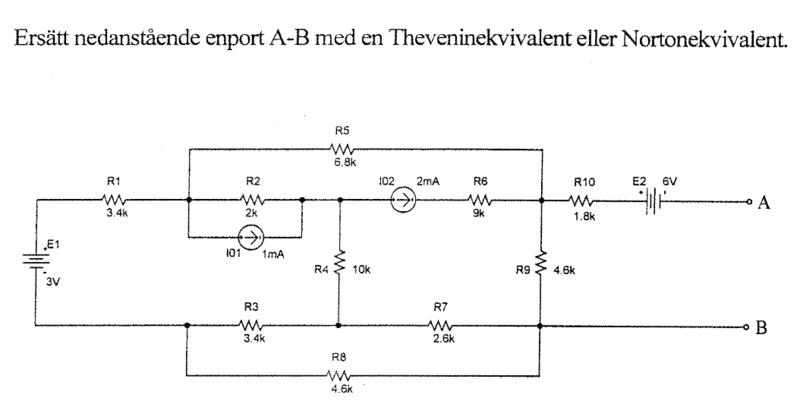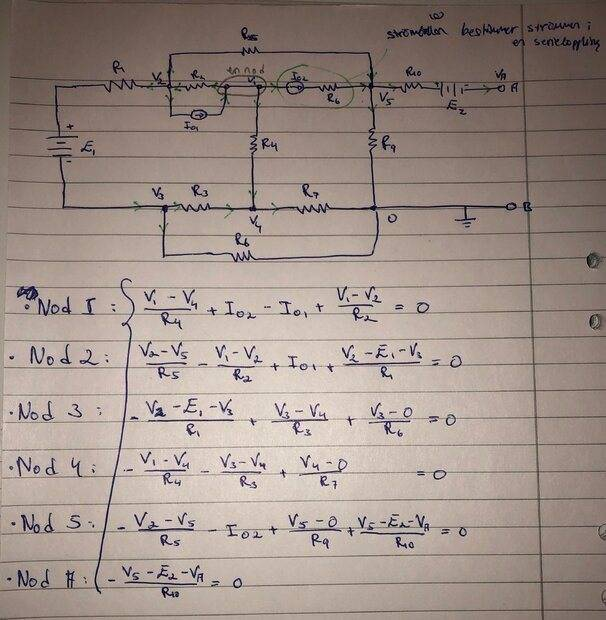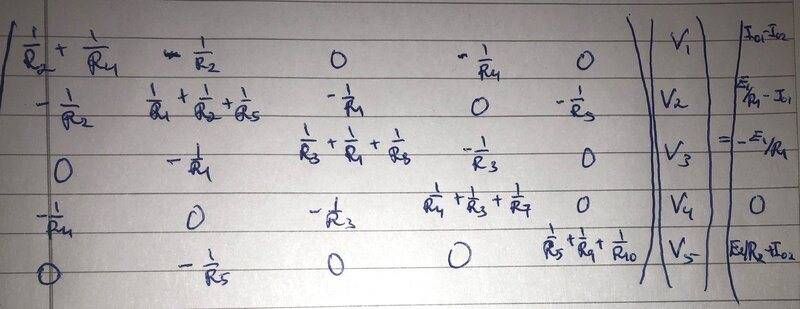Gold Member
What are the real voltages? Where did they come from? Did the problem statement include the answers, or did the professor or a classmate of yours provide them?

In the last row of the stimulus vector you have E2/R2+Io2 (or so it appears); why do you have R2 in the denominator?

David331
Thanks for the response! I got the real answers using multisim:
--- Operating Point ---
V(n001): 0.683191 voltage
V(3): -2.31681 voltage
V(2): -2.01304 voltage
V(1): -3.56637 voltage
V(4): -1.333 voltage
V(5): 4.6758 voltage
V(n002): 22.6758 voltage
V(n003): 4.67604 voltage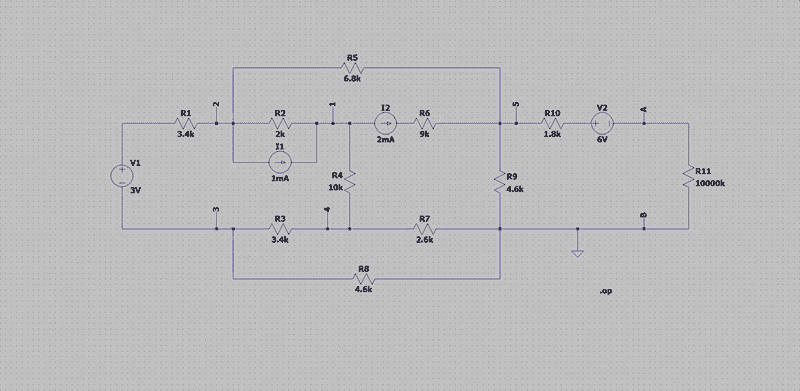I see that I have written a R2 instead of R10, but I have used R10 when I have made my calculations. Does the calculations and equationsystem look correct though?

Gold Member
Thanks for the response! I got the real answers using multisim:
--- Operating Point ---
V(n001): 0.683191 voltage
V(3): -2.31681 voltage
V(2): -2.01304 voltage
V(1): -3.56637 voltage
V(4): -1.333 voltage
V(5): 4.6758 voltage
V(n002): 22.6758 voltage
V(n003): 4.67604 voltage
View attachment 289513

I see that I have written a R2 instead of R10, but I have used R10 when I have made my calculations. Does the calculations and equationsystem look correct though?
The circuit you solved with multisim has a 10000k resistor, R11, that your circuit in post #1 does not have. If you include that resistor you will get the same result as multisim: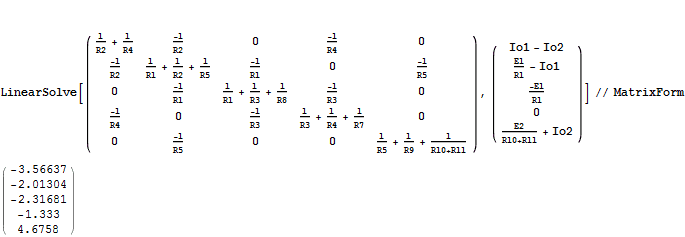David331
Ohhh I did not know that R11 affected the results, only used it for easier simulation. I am so thankful for the help, I have been stuck on this assignment very long! So now to get the open circuit voltage I have to take U0=VA-VB=VA-0=VA=V5-E2=5,53088-6,00000=-1,3242 voltage. And to get the short-circuit current I take I0=(V5-E2-0)/R10=(5,53088-6,00000)/1800=-0,2606...mA?

Gold Member
Ohhh I did not know that R11 affected the results, only used it for easier simulation. I am so thankful for the help, I have been stuck on this assignment very long! So now to get the open circuit voltage I have to take U0=VA-VB=VA-0=VA=V5-E2=5,53088-6,00000=-1,3242 voltage. And to get the short-circuit current I take I0=(V5-E2-0)/R10=(5,53088-6,00000)/1800=-0,2606...mA?
You have the short circuit current correct, but for the open circuit voltage you need the voltage at node 5 without R10 and E2 connected from node 5 to ground. It's easy to delete R10 from the 5,5 element of the matrix in post #1 and from the 5,1 element of the corresponding stimulus vector and solve. What do you get if you do that?

David331
In that case I get
V =

-3.566523917173452 (V1)
-2.013218390804596 (V2)
-2.316892200252596 (V3)
-1.333051549017734 (V4)
4.675368017745513 (V5)
Which is the same as the simulation gave.
In this case I get U0=V5-E2=4.675368...-6.000000=-1.324632 V.(I see that I got this in last also, but computing something completely else...). So this is the correct open circuit voltage? How come we don't need E2 and R10 in our calculations?

Gold Member
In that case I get
V =

-3.566523917173452 (V1)
-2.013218390804596 (V2)
-2.316892200252596 (V3)
-1.333051549017734 (V4)
4.675368017745513 (V5)
Which is the same as the simulation gave.
In this case I get U0=V5-E2=4.675368...-6.000000=-1.324632 V.(I see that I got this in last also, but computing something completely else...). So this is the correct open circuit voltage? How come we don't need E2 and R10 in our calculations?
These voltages are not exactly the same as the simulation; R11 does have a very small effect.

If the A-B port is open circuited then the right end of E2 should not be grounded. The matrix you used in post #1 has the right end of E2 grounded (A-B shorted). But once you have the voltage at node 5 with R10 and E2 not grounded (A-B open circuited), the voltage at A is just the same as the voltage at node 5 minus the voltage of E2 since there is no voltage drop across R10; R10 carries no current if the right end of E2 is floating.

David331
Oh now I see, I did not think about that there was no voltage drop across R10, since as you say, no current flows through when it is open circuited. Thanks a lot for the explantions, I have learned much! Just to clarify, the U0 voltage is now correct right?

Gold Member
Oh now I see, I did not think about that there was no voltage drop across R10, since as you say, no current flows through when it is open circuited. Thanks a lot for the explantions, I have learned much! Just to clarify, the U0 voltage is now correct right?
Provided you don't use the value of V5 from post #3, but rather from the procedure I described in post #6.

David331
Yes of course, now I used the values computed in #7 (V5=4.675368017745513 V), which indeed differ a bit from the values in the simulation.

Gold Member
Yes of course, now I used the values computed in #7 (V5=4.675368017745513 V), which indeed differ a bit from the values in the simulation.
Good luck with the rest of your studies.
You might find post #62 here interesting showing use of nodal analysis: https://www.physicsforums.com/goto/post?id=6421623

•David331
David331
Thanks and once again thanks for all the help! I will save that link for later use, as I progress throughout my engineering courses, it will definitely be helpful :)